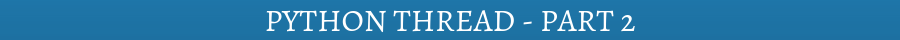Python thread is like a process, and may even be a process, depending on the Python thread system. In fact, Python threads are sometimes called “lightweight” processes, because threads occupy much less memory, and take less time to create than do processes.

In python, threading module is used to create the threads. In order to create threads, threading modules can be used in two ways. First, by inheriting the class and second by the use of thread function.

Python Thread creation using class :

Let us understand by using code.

def __init__(self,i):

self.h =i

def run(self):

print "Value send ", self.h

• __init__(self [,args]) Override the constructor
• run():  This is the section where you can put your logic part
• start(): The start() method starts a Python thread
• The mythread class overrides the constructor, so base class constructor (Thread.__init__()) must be invoked.

Python Thread creation using function :

def fun1(a,b):

c = a+b

print (c)

The above code is very simple, just create a function and get it run by a thread. The syntax

specifies the function to be run and args indicates the tuple which contains the argument to be passed to the function.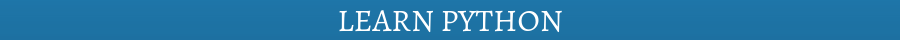Let us see some important methods, defined in the threading modules.

Let us understand by the example.

import time

def fun1(a,b):

time.sleep(1)

c = a+b

print (c)

Let us see the output.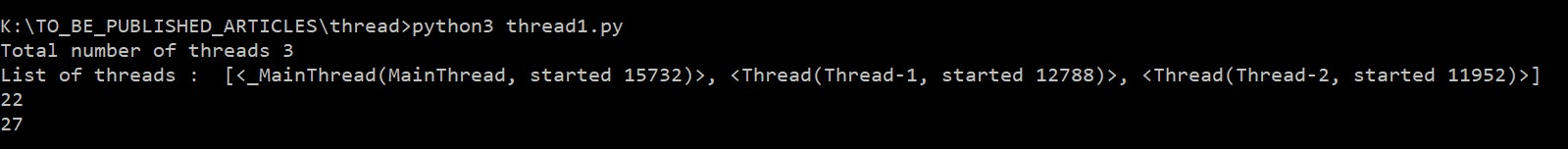Now comment the line time.sleep(1) and then run the code again.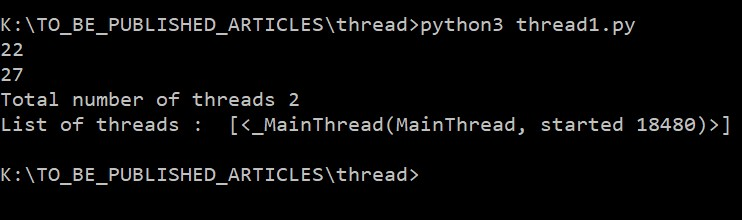Figure 2:  Without sleep time

You can see the difference. The statement threading.activeCount() and threading.enumerate() have been run the main thread or main process. So, the main thread is responsible to run the entire program. In figure 1 when main thread executed the threading.activeCount(), that time thread1 and thread2 were active.

When we commented the time.sleep(1) syntax, and executed the code again then thread1 and thread3 might not be active.

The join method

Before discussing the significance of join method, let us see the following program.

import time

list1 = []

def fun1(a):

time.sleep(1) # complex calculation takes 1 seconds

list1.append(a)

print ("List1 is : ", list1)

In the above program, the two threads have been created with arguments. In target function fun1, a global list list1 is appended with an argument. Let us see the result.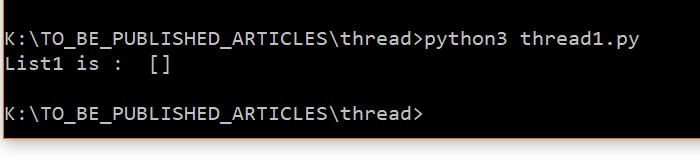Figure 3: Showing Empty list

The above figure is showing the empty list, but the list is expected to be filled with values 1 and 6. The statement print (“List1 is : “, list1) is executed by the main thread and main thread printed the list before getting it filled.

So main thread must be paused until all threads complete their jobs. To achieve the same, we shall use the join method.

Let see the modified code.

import time

list1 = []

def fun1(a):

time.sleep(1) # complex calculation takes 1 seconds

list1.append(a)

print ("List1 is : ", list1)

Let us see the output.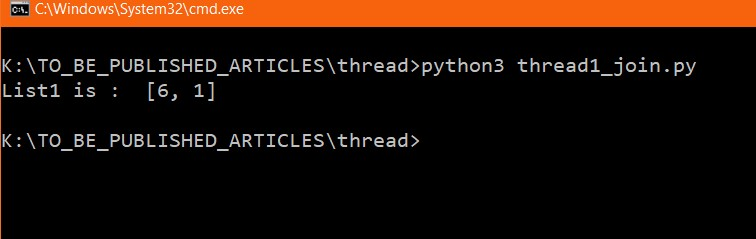Figure 4: use of join method

In the above code the syntax thread1.join() block the main thread until thread1 finishes its task. In order to achieve parallelism, join method must be called after creation of all threads.

Let us see more use case of join methods.

import time

import datetime

t1 = datetime.datetime.now()

list1 = []

def fun1(a):

time.sleep(1) # complex calculation takes 1 seconds

list1.append(a)

for each in range(10):

th.join()

print ("List1 is : ", list1)

t2 = datetime.datetime.now()

print ("Time taken", t2-t1)

In the above code, we have used for loop to create 10 threads. Each thread is getting appended in the list list_thread. After creation of all threads, we used the join method. Let us see the output.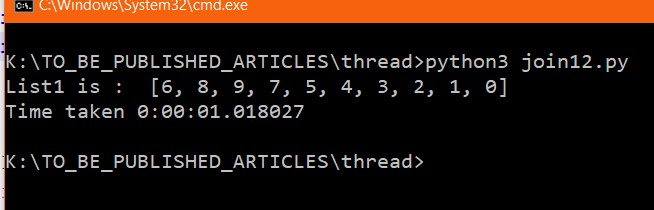Figure 5: Out of join with loop

Time taken to execute is approx. 1 second. Since every thread take 1 second and we used thread-based parallelism that’s why total time is near to 1 second. If you use the join method after the creation of each thread program would take more than 10 seconds.

For more clarification see the following code.

import time

import datetime

t1 = datetime.datetime.now()

list1 = []

def fun1(a):

time.sleep(1) # complex calculation takes 1 seconds

list1.append(a)

for each in range(10):

print ("List1 is : ", list1)

t2 = datetime.datetime.now()

print ("Time taken", t2-t1)

Output: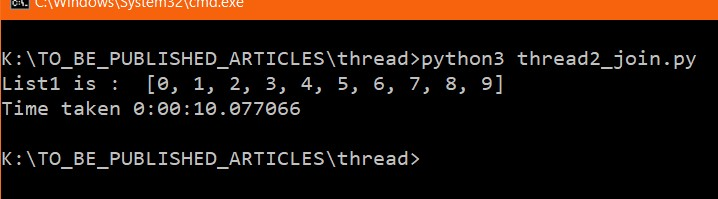Figure 6: Thread with join method

From the above output, it is clear that, time taken is 10 seconds, it means no parallelism has been achieved because Join() method of first thread has been called before the creation of the second thread.

Method join with time

Let us see the following piece of code.

import time

def fun1(a):

time.sleep(3) # complex calculation takes 3 seconds

The couples of things are new here. The isAlive() method returns the True or False. If the thread is currently active then the method isAlive() returns True otherwise False.

Output: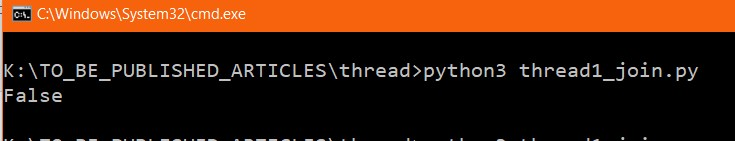The above output shows thread is not active.

Let make a small change, make thread1.join() to thread1.join(2). It means, block the main thread only for 2 seconds.

Let us see the output: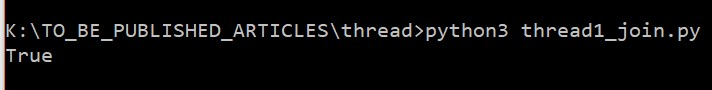In the above output, show thread was still active because join(2) kept blocking the main thread for 2 seconds but the thread took 3 seconds to complete its task.